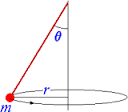# Circular motion lab problem

## Homework Statement

For a conical pendulum:

Construct a free-body diagram for the pendulum and derive an expression for the acceleration in terms of the gravitational field strength g, the length L of the pendulum, the radius r of the circular path of the pendulum, and the frequency f of oscillation. (Hint - first derive an expression for tanθ the angle between the string and the vertical.)a=v^2/r
a=Δv/Δt
a=ƩF/m

## The Attempt at a Solution

Have done free body diagram, can't figure out how to make equation.

Last edited:

## Answers and Replies

haruspex
Science Advisor
Homework Helper
Gold Member
2020 Award
There is a specific format you are supposed to use on this forum.
What do you have for the forces - just describe them, don't worry about a diagram.
What do you have for the acceleration?

Thank you for replying; didn't see I had to use that form.
As for forces there is the force of gravity on the pendulum mass (downwards), and the tension in the string.
As for the acceleration - I have no numerical value for it. The question needs no actual values - just variables to make the equation (a in terms of g, L, r, and f).

haruspex
Science Advisor
Homework Helper
Gold Member
2020 Award
Thank you for replying; didn't see I had to use that form.
As for forces there is the force of gravity on the pendulum mass (downwards), and the tension in the string.
As for the acceleration - I have no numerical value for it. The question needs no actual values - just variables to make the equation (a in terms of g, L, r, and f).
True, but you do know the direction the acceleration has to be in. So in what directions would you resolve the forces, and what equations result?